GeeksforGeeks App
Open AppBrowser
Continue

In this article, we are going to see how to change the color of boxplots using ggplot2 in R Programming Language.

We have considered the built-in data frame “ChickWeight”. It contains information about the feed type and growth rate of chickens for six different types of foods like casein, soybean, etc.  It has two vectors feed which will be in the x-axis and weight which will be in the y-axis.

### Setting Default colors

• Same outlines

Here we will use the color keyword. Outliers are observations that are located outside the whiskers of a box plot. We will keep the default black color for them. Use the command outlier.color to add color to the outliers in the plot. Since we need the same color in the outlines, we will write the command inside the geom_boxplot( ).

Example:

## R

 `# Same outline color``library``(ggplot2)` `ch <- ``ggplot``(chickwts,``aes``(x=feed,y=weight))+``      ``geom_boxplot``(color=``"red"``,outlier.color=``"black"``)` `ch`

Output: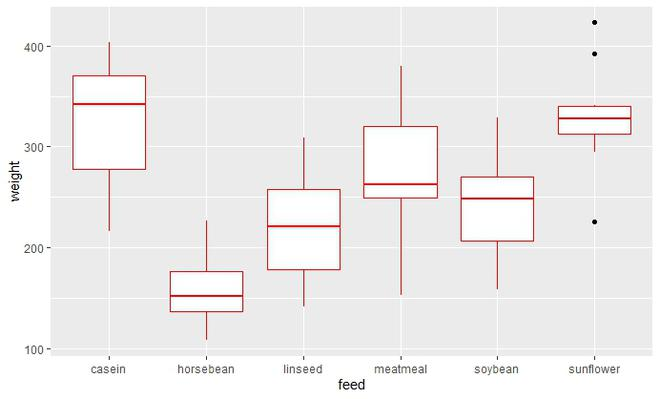• Different outlines

Since the color is variable here, we will write the command color inside aes( ) of ggplot assigned to the x-axis value. The outliers will have the same black color, so write the command inside the geom_boxplot( ) only.

Example:

## R

 `# Different outline color``library``(ggplot2)` `ch <- ``ggplot``(chickwts,``aes``(x=feed,y=weight,color=feed))+``      ``geom_boxplot``(outlier.color=``"black"``)` `ch`

Output: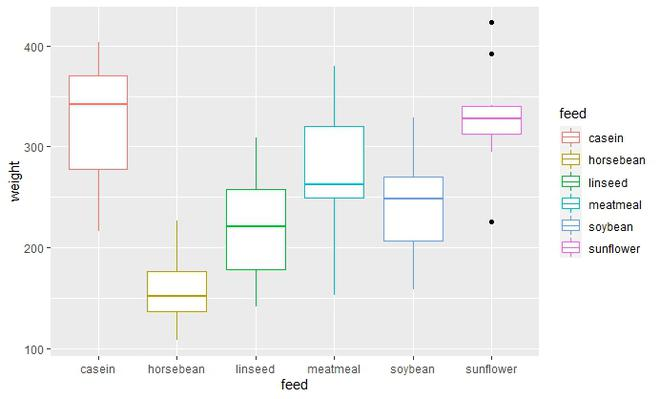• Same fill

Here, we will use the keyword fill. Since we need the same color in the fill of boxplots, we will write the command inside the geom_boxplot( ). By default, fill for outliers is black.

Example:

## R

 `# Same fill color``library``(ggplot2)` `ch <- ``ggplot``(chickwts,``aes``(x=feed,y=weight))+``      ``geom_boxplot``(fill=``"violet"``)` `ch`

Output: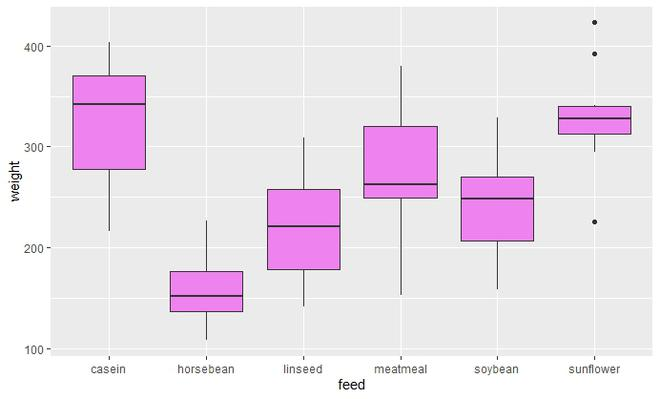• Different fill

Since the color of fill is variable here, we will write the command fill inside aes( ) of ggplot assigned to the x-axis value.

Example:

## R

 `# Different fill color``library``(ggplot2)` `ch <- ``ggplot``(chickwts,``aes``(x=feed,y=weight,fill=feed))+``      ``geom_boxplot``()` `ch`

Output: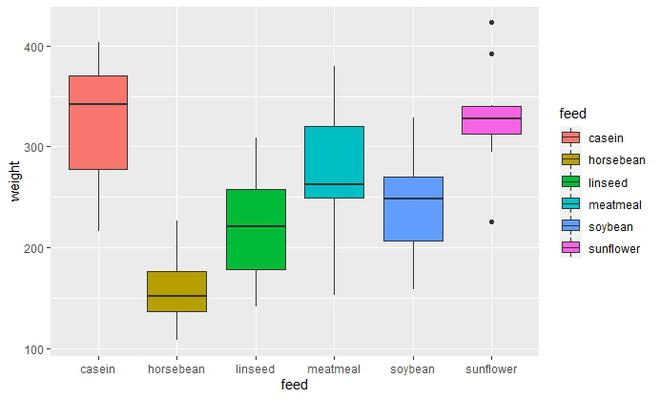### Setting color manually

1) For outlines

In this we use the following functions :

• scale_color_manual( ) : Custom colors available. You can either enter the color code using “#XXXXXX” or simply write the color name.

Syntax:

scale_color_manual( values)

• scale_color_brewer( ) : Tons of color palettes are available in the RColorBrewer package.

Syntax:

scale_color_brewer(palette)

• scale_color_grey( ) : It is used to add gray scale.

Example:

## R

 `library``(ggplot2)` `ch <- ``ggplot``(chickwts,``aes``(x=feed,y=weight,color=feed))+``      ``geom_boxplot``(outlier.colour = ``"black"``)` `# Assign custom color``ch+``scale_color_manual``(values=``c``(``"#999999"``,``                               ``"purple"``,``                               ``"#33FFFF"``,``                               ``"red"``,``                               ``"green"``,``                               ``"brown"``))``# Assign brewer color``ch+``scale_color_brewer``(palette=``"Dark2"``)` `# Assign gray scale``ch+``scale_color_grey``() + ``theme_classic``()`

Output: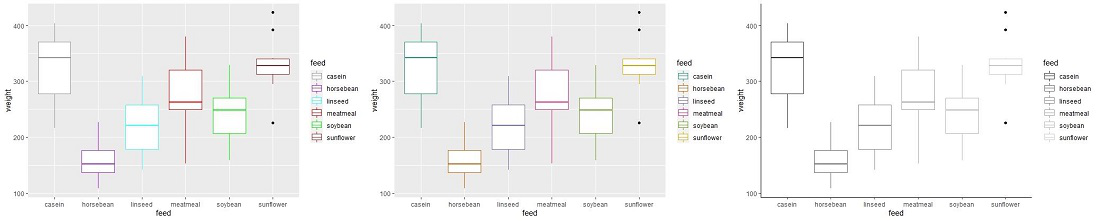2) For filling

Similarly, we can fill the box plot. The functions used for this are:

• scale_fill_manual( ) Custom colors available. You can either enter the color code using “#XXXXXX” or simply write the color name.

Syntax:

scale_fill_manual( values)

• scale_fill_brewer( ) : Tons of color palettes are available in the RColorBrewer package.

Syntax:

scale_fill_brewer(palette)

• scale_fill_grey( ) : It is used to add gray scale.

Example:

## R

 `library``(ggplot2)` `ch <- ``ggplot``(chickwts,``aes``(x=feed,y=weight,fill=feed))+``      ``geom_boxplot``()` `ch` `# Assign custom color``ch+``scale_fill_manual``(values=``c``(``"#999999"``,``                               ``"purple"``,``                               ``"#33FFFF"``,``                               ``"red"``,``                               ``"green"``,``                               ``"brown"``))` `# Assign brewer color``ch+``scale_fill_brewer``(palette=``"Dark2"``)` `# Assign gray scale``ch+``scale_fill_grey``() + ``theme_classic``()`

Output: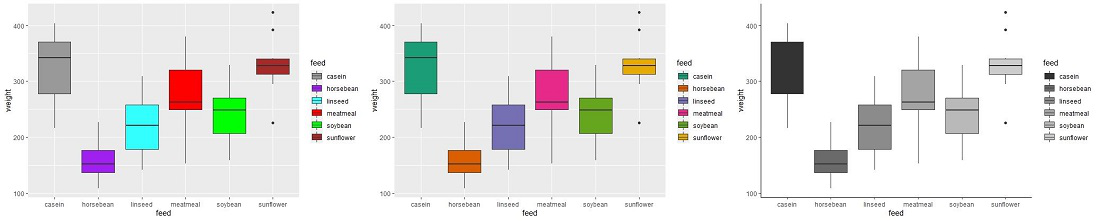My Personal Notes arrow_drop_up